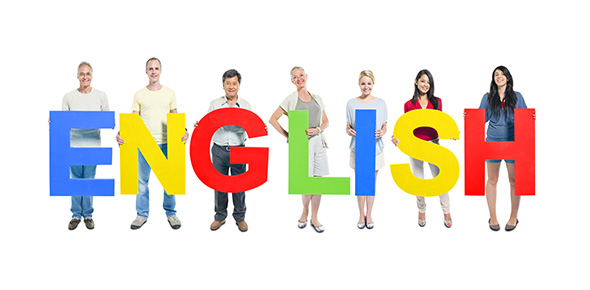# A Trivia Quiz On Letter Recognition!

Approved & Edited by ProProfs Editorial Team
The editorial team at ProProfs Quizzes consists of a select group of subject experts, trivia writers, and quiz masters who have authored over 10,000 quizzes taken by more than 100 million users. This team includes our in-house seasoned quiz moderators and subject matter experts. Our editorial experts, spread across the world, are rigorously trained using our comprehensive guidelines to ensure that you receive the highest quality quizzes.
| By Mlpeters
M
Mlpeters
Community Contributor
Quizzes Created: 1 | Total Attempts: 151
Questions: 10 | Attempts: 151SettingsThis Quiz is about Letter Recognition. Students will be assisted by an adult to fill out the quiz. Students will point to their answer on the screen and the adult will click on the answer they chose. So, let's try out the quiz. All the best!

• 1.

### Point to the capital letter "H".

• A.

H

• B.

B

• C.

'h'

• D.

T

A. H
Explanation
The correct answer is "H" because it is the only capital letter in the given options.

Rate this question:

• 2.

### Point to the capital letter "K".

• A.

N

• B.

F

• C.

'k'

• D.

K

D. K
Explanation
The correct answer is K because it is the only capital letter among the given options.

Rate this question:

• 3.

### Point to the lowercase letter "B".

• A.

D

• B.

B

• C.

'b'

• D.

D

C. 'b'
Explanation
The lowercase letter "B" is pointed to by the answer "b".

Rate this question:

• 4.

### Point to the capital letter "N".

• A.

M

• B.

'n'

• C.

H

• D.

N

D. N
Explanation
The correct answer is "N" because the question asks to point to the capital letter "N" and the only capital letter in the given options is "N".

Rate this question:

• 5.

### Point to the lowercase letter "H".

• A.

A

• B.

'h'

• C.

H

• D.

U

C. H
Explanation
The correct answer is "H" because it is the only lowercase letter among the options provided.

Rate this question:

• 6.

### Point to the lowercase letter "K".

• A.

'k'

• B.

W

• C.

I

• D.

M

A. 'k'
Explanation
The correct answer is 'k' because it is the only lowercase letter among the given options.

Rate this question:

• 7.

### Point to the lowercase letter "D".

• A.

B

• B.

'd'

• C.

D

• D.

B

B. 'd'
Explanation
The correct answer is 'd' because it is the lowercase letter that is being pointed to in the given options.

Rate this question:

• 8.

### Point to the lowercase letter "N".

• A.

M

• B.

N

• C.

M

• D.

'n'

D. 'n'
Explanation
The correct answer is "n" because it is the only lowercase letter among the options given. The other letters, M, m, and 'n', are either uppercase or special characters.

Rate this question:

• 9.

### Point to the lowercase letter "I".

• A.

'i'

• B.

L

• C.

J

• D.

E

A. 'i'
Explanation
The lowercase letter "I" is represented by the letter "i" in the given options. It is the second letter in the first row.

Rate this question:

• 10.

### Point to the lowercase letter "J".

• A.

J

• B.

I

• C.

T

• D.

'j'

D. 'j'
Explanation
The correct answer is "j" because it is the only lowercase letter in the given options.

Rate this question:

Related TopicsBack to top